Documentation

# binScatterPlot

Scatter plot of bins for tall arrays

## Syntax

``binScatterPlot(X,Y)``
``binScatterPlot(X,Y,nbins)``
``binScatterPlot(X,Y,Xedges,Yedges)``
``binScatterPlot(X,Y,Name,Value)``
``h = binScatterPlot(___)``

## Description

example

````binScatterPlot(X,Y)` creates a binned scatter plot of the data in `X` and `Y`. The `binScatterPlot` function uses an automatic binning algorithm that returns bins with a uniform area, chosen to cover the range of elements in `X` and `Y` and reveal the underlying shape of the distribution.```

example

````binScatterPlot(X,Y,nbins)` specifies the number of bins to use in each dimension.```

example

````binScatterPlot(X,Y,Xedges,Yedges)` specifies the edges of the bins in each dimension using the vectors `Xedges` and `Yedges`.```

example

````binScatterPlot(X,Y,Name,Value)` specifies additional options with one or more name-value pair arguments using any of the previous syntaxes. For example, you can specify `'Color'` and a valid color option to change the color theme of the plot, or `'Gamma'` with a positive scalar to adjust the level of detail.```
````h = binScatterPlot(___)` returns a `Histogram2` object. Use this object to inspect properties of the plot.```

## Examples

collapse all

Create two tall vectors of random data. Create a binned scatter plot for the data.

`X = tall(randn(1e5,1));`
```Starting parallel pool (parpool) using the 'local' profile ... Connected to the parallel pool (number of workers: 4). ```
`Y = tall(randn(1e5,1));`

When you perform calculations on tall arrays, MATLAB® uses either a parallel pool (default if you have Parallel Computing Toolbox™) or the local MATLAB session. If you want to run the example using the local MATLAB session when you have Parallel Computing Toolbox, you can change the global execution environment by using the `mapreducer` function.

`binScatterPlot(X,Y)````Evaluating tall expression using the Parallel Pool 'local': - Pass 1 of 1: Completed in 5.7 sec Evaluation completed in 9.4 sec Evaluating tall expression using the Parallel Pool 'local': - Pass 1 of 1: Completed in 1.8 sec Evaluation completed in 2.9 sec ```

The resulting figure contains a slider to adjust the level of detail in the image.

Specify a scalar value as the third input argument to use the same number of bins in each dimension, or a two-element vector to use a different number of bins in each dimension.

Plot a binned scatter plot of random data sorted into 100 bins in each dimension.

`X = tall(randn(1e5,1));`
```Starting parallel pool (parpool) using the 'local' profile ... Connected to the parallel pool (number of workers: 4). ```
`Y = tall(randn(1e5,1));`

When you perform calculations on tall arrays, MATLAB® uses either a parallel pool (default if you have Parallel Computing Toolbox™) or the local MATLAB session. If you want to run the example using the local MATLAB session when you have Parallel Computing Toolbox, you can change the global execution environment by using the `mapreducer` function.

`binScatterPlot(X,Y,100)`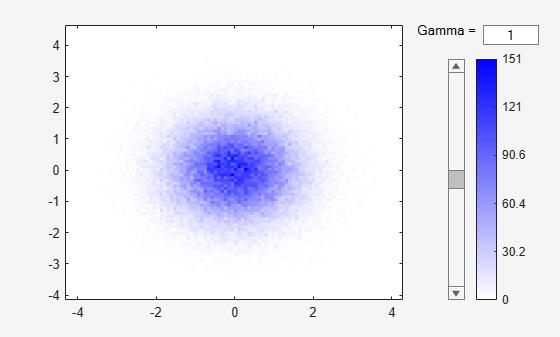```Evaluating tall expression using the Parallel Pool 'local': - Pass 1 of 1: Completed in 3.6 sec Evaluation completed in 5.7 sec Evaluating tall expression using the Parallel Pool 'local': - Pass 1 of 1: Completed in 2.5 sec Evaluation completed in 3.5 sec ```

Use 20 bins in the x-dimension and continue to use 100 bins in the y-dimension.

`binScatterPlot(X,Y,[20 100])`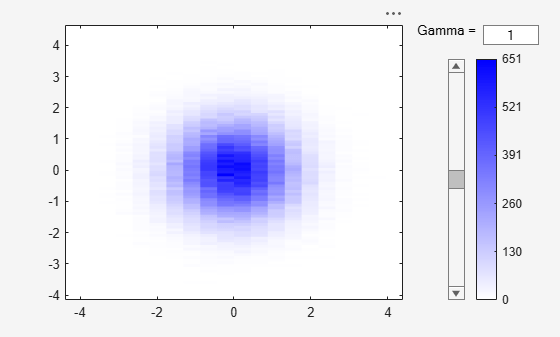```Evaluating tall expression using the Parallel Pool 'local': - Pass 1 of 1: Completed in 1.6 sec Evaluation completed in 2.2 sec Evaluating tall expression using the Parallel Pool 'local': - Pass 1 of 1: Completed in 0.71 sec Evaluation completed in 1.5 sec ```

Plot a binned scatter plot of random data with specific bin edges. Use bin edges of `Inf` and `-Inf` to capture outliers.

Create a binned scatter plot with 100 bin edges between `[-2 2]` in each dimension. The data outside the specified bin edges is not included in the plot.

`X = tall(randn(1e5,1));`
```Starting parallel pool (parpool) using the 'local' profile ... Connected to the parallel pool (number of workers: 4). ```
`Y = tall(randn(1e5,1));`

When you perform calculations on tall arrays, MATLAB® uses either a parallel pool (default if you have Parallel Computing Toolbox™) or the local MATLAB session. If you want to run the example using the local MATLAB session when you have Parallel Computing Toolbox, you can change the global execution environment by using the `mapreducer` function.

```Xedges = linspace(-2,2); Yedges = linspace(-2,2); binScatterPlot(X,Y,Xedges,Yedges)```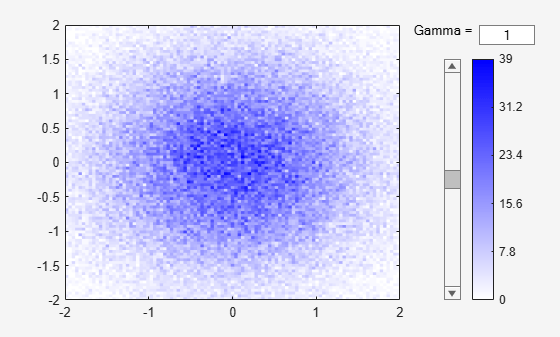```Evaluating tall expression using the Parallel Pool 'local': - Pass 1 of 1: Completed in 4.7 sec Evaluation completed in 7.1 sec ```

Use coarse bins extending to infinity on the edges of the plot to capture outliers.

```Xedges = [-Inf linspace(-2,2) Inf]; Yedges = [-Inf linspace(-2,2) Inf]; binScatterPlot(X,Y,Xedges,Yedges)```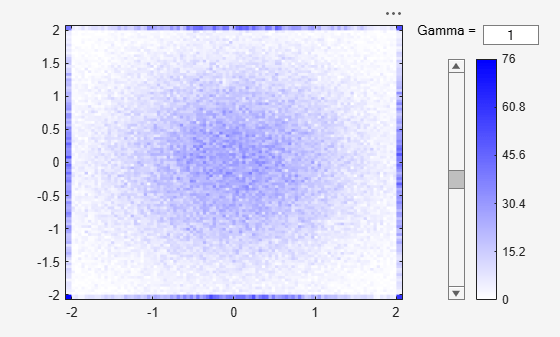```Evaluating tall expression using the Parallel Pool 'local': - Pass 1 of 1: Completed in 2.1 sec Evaluation completed in 2.7 sec ```

Plot a binned scatter plot of random data, specifying `'Color'` as `'c'`.

`X = tall(randn(1e5,1));`
```Starting parallel pool (parpool) using the 'local' profile ... Connected to the parallel pool (number of workers: 4). ```
`Y = tall(randn(1e5,1));`

When you perform calculations on tall arrays, MATLAB® uses either a parallel pool (default if you have Parallel Computing Toolbox™) or the local MATLAB session. If you want to run the example using the local MATLAB session when you have Parallel Computing Toolbox, you can change the global execution environment by using the `mapreducer` function.

`binScatterPlot(X,Y,'Color','c')`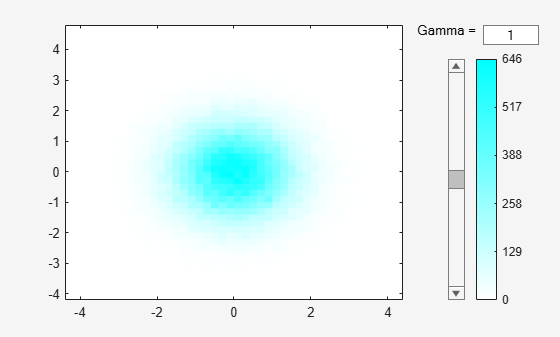```Evaluating tall expression using the Parallel Pool 'local': - Pass 1 of 1: Completed in 4.2 sec Evaluation completed in 6.6 sec Evaluating tall expression using the Parallel Pool 'local': - Pass 1 of 1: Completed in 1.8 sec Evaluation completed in 2.8 sec ```

## Input Arguments

collapse all

Data to distribute among bins, specified as separate arguments of tall vectors, matrices, or multidimensional arrays. `X` and `Y` must be the same size. If `X` and `Y` are not vectors, then `binScatterPlot` treats them as single column vectors, `X(:)` and `Y(:)`.

Corresponding elements in `X` and `Y` specify the x and y coordinates of 2-D data points, `[X(k),Y(k)]`. The underlying data types of `X` and `Y` can be different, but `binScatterPlot` concatenates these inputs into a single `N`-by-`2` tall matrix of the dominant underlying data type.

`binScatterPlot` ignores all `NaN` values. Similarly, `binScatterPlot` ignores `Inf` and `-Inf` values, unless the bin edges explicitly specify `Inf` or `-Inf` as a bin edge.

### Note

If `X` or `Y` contain integers of type `int64` or `uint64` that are larger than `flintmax`, then it is recommended that you explicitly specify the bin edges.`binScatterPlot` automatically bins the input data using double precision, which lacks integer precision for numbers greater than `flintmax`.

Data Types: `single` | `double` | `int8` | `int16` | `int32` | `int64` | `uint8` | `uint16` | `uint32` | `uint64` | `logical`

Number of bins in each dimension, specified as a positive scalar integer or two-element vector of positive integers. If you do not specify `nbins`, then `binScatterPlot` automatically calculates how many bins to use based on the values in `X` and `Y`.

• If `nbins` is a scalar, then `binScatterPlot` uses that many bins in each dimension.

• If `nbins` is a vector, then `nbins(1)` specifies the number of bins in the x-dimension and `nbins(2)` specifies the number of bins in the y-dimension.

Example: `binScatterPlot(X,Y,20)` uses 20 bins in each dimension.

Example: ```binScatterPlot(X,Y,[10 20])``` uses 10 bins in the `x`-dimension and 20 bins in the `y`-dimension.

Bin edges in x-dimension, specified as a vector. `Xedges(1)` is the first edge of the first bin in the x-dimension, and `Xedges(end)` is the outer edge of the last bin.

The value `[X(k),Y(k)]` is in the `(i,j)`th bin if `Xedges(i)``X(k)` < `Xedges(i+1)` and `Yedges(j)``Y(k)` < `Yedges(j+1)`. The last bins in each dimension also include the last (outer) edge. For example, `[X(k),Y(k)]` falls into the `i`th bin in the last row if `Xedges(end-1)``X(k)``Xedges(end)` and `Yedges(i)``Y(k)` < `Yedges(i+1)`.

Data Types: `single` | `double` | `int8` | `int16` | `int32` | `int64` | `uint8` | `uint16` | `uint32` | `uint64` | `logical`

Bin edges in y-dimension, specified as a vector. `Yedges(1)` is the first edge of the first bin in the y-dimension, and `Yedges(end)` is the outer edge of the last bin.

The value `[X(k),Y(k)]` is in the `(i,j)`th bin if `Xedges(i)``X(k)` < `Xedges(i+1)` and `Yedges(j)``Y(k)` < `Yedges(j+1)`. The last bins in each dimension also include the last (outer) edge. For example, `[X(k),Y(k)]` falls into the `i`th bin in the last row if `Xedges(end-1)``X(k)``Xedges(end)` and `Yedges(i)``Y(k)` < `Yedges(i+1)`.

Data Types: `single` | `double` | `int8` | `int16` | `int32` | `int64` | `uint8` | `uint16` | `uint32` | `uint64` | `logical`

### Name-Value Pair Arguments

Specify optional comma-separated pairs of `Name,Value` arguments. `Name` is the argument name and `Value` is the corresponding value. `Name` must appear inside quotes. You can specify several name and value pair arguments in any order as `Name1,Value1,...,NameN,ValueN`.

Example: `binScatterPlot(X,Y,'BinWidth',[5 10])`

Binning algorithm, specified as the comma-separated pair consisting of `'BinMethod'` and one of these values.

ValueDescription
`'auto'`The default `'auto'` algorithm uses a maximum of 100 bins and chooses a bin width to cover the data range and reveal the shape of the underlying distribution.
`'scott'`Scott’s rule is optimal if the data is close to being jointly normally distributed. This rule is appropriate for most other distributions, as well. It uses a bin size of ```[3.5*std(X)*numel(X)^(-1/4), 3.5*std(Y)*numel(Y)^(-1/4)]```.
`'integers'`The integer rule is useful with integer data, as it creates a bin for each integer. It uses a bin width of 1 and places bin edges halfway between integers. To avoid accidentally creating too many bins, you can use this rule to create a limit of 65536 bins (216). If the data range is greater than 65536, then the integer rule uses wider bins instead.

### Note

The `BinMethod` property of the resulting `Histogram2` object always has a value of `'manual'`.

Width of bins in each dimension, specified as the comma-separated pair consisting of `'BinWidth'` and a scalar or two-element vector of positive integers, `[xWidth yWidth]`. A scalar value indicates the same bin width for each dimension.

If you specify `BinWidth`, then `binScatterPlot` can use a maximum of 1024 bins (210) along each dimension. If instead the specified bin width requires more bins, then `binScatterPlot` uses a larger bin width corresponding to the maximum number of bins.

Example: `binScatterPlot(X,Y,'BinWidth',[5 10])` uses bins with size `5` in the `x`-dimension and size `10` in the `y`-dimension.

Plot color theme, specified as the comma-separated pair consisting of `'Color'` and one of these options.

OptionDescription
`'b'`

Blue

`'m'`

Magenta

`'c'`

Cyan

`'r'`

Red

`'g'`

Green

`'y'`

Yellow

`'k'`

Black

Gamma correction, specified as the comma-separated pair consisting of `'Gamma'` and a positive scalar. Use this option to adjust the brightness and color intensity to affect the amount of detail in the image.

• `gamma < 1` — As gamma decreases, the shading of bins with smaller bin counts becomes progressively darker, including more detail in the image.

• `gamma > 1` — As gamma increases, the shading of bins with smaller bin counts becomes progressively lighter, removing detail from the image.

• The default value of 1 does not apply any correction to the display.

Bin limits in x-dimension, specified as the comma-separated pair consisting of `'XBinLimits'` and a two-element vector, `[xbmin,xbmax]`. The vector indicates the first and last bin edges in the x-dimension.

`binScatterPlot` only plots data that falls within the bin limits inclusively, ```Data(Data(:,1)>=xbmin & Data(:,1)<=xbmax)```.

Bin limits in y-dimension, specified as the comma-separated pair consisting of `'YBinLimits'` and a two-element vector, `[ybmin,ybmax]`. The vector indicates the first and last bin edges in the y-dimension.

`binScatterPlot` only plots data that falls within the bin limits inclusively, ```Data(Data(:,2)>=ybmin & Data(:,2)<=ybmax)```.

## Output Arguments

collapse all

Binned scatter plot, returned as a `Histogram2` object. For more information, see Histogram2 Properties.